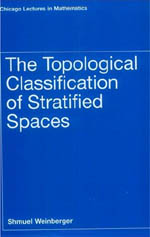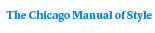# The Topological Classification of Stratified Spaces

Shmuel Weinberger298 pages | 15 line drawings | 6 x 9 | © 1994
Paper \$34.00 ISBN: 9780226885674 Published January 1995
Cloth \$87.00 ISBN: 9780226885667 Published February 1995
This book provides the theory for stratified spaces, along with important examples and applications, that is analogous to the surgery theory for manifolds. In the first expository account of this field, Weinberger provides topologists with a new way of looking at the classification theory of singular spaces with his original results.

Divided into three parts, the book begins with an overview of modern high-dimensional manifold theory. Rather than including complete proofs of all theorems, Weinberger demonstrates key constructions, gives convenient formulations, and shows the usefulness of the technology. Part II offers the parallel theory for stratified spaces. Here, the topological category is most completely developed using the methods of "controlled topology." Many examples illustrating the topological invariance and noninvariance of obstructions and characteristic classes are provided. Applications for embeddings and immersions of manifolds, for the geometry of group actions, for algebraic varieties, and for rigidity theorems are found in Part III.

This volume will be of interest to topologists, as well as mathematicians in other fields such as differential geometry, operator theory, and algebraic geometry.
Table of Contents• Contents
Contents
Introduction
Part I: Manifold theory
1. Algebraic K-theory and topology
2. Surgery theory
3. Spacification and functoriality
4. Applications
Part II: General theory
5. Definitions and examples
6. Classification of stratified spaces
7. Transverse stratified classification
8. PT category
9. Controlled topology
10. Proof of main theorems in topology
Part III: Applications and illustrations
11. Manifolds and embedding theory revisited
12. Supernormal spaces and varieties
13. Group actions
14. Rigidity conjectures
Bibliography
Index